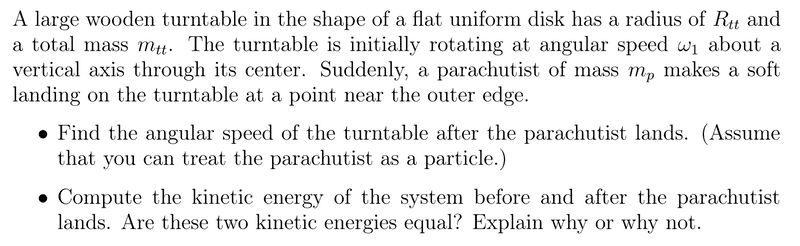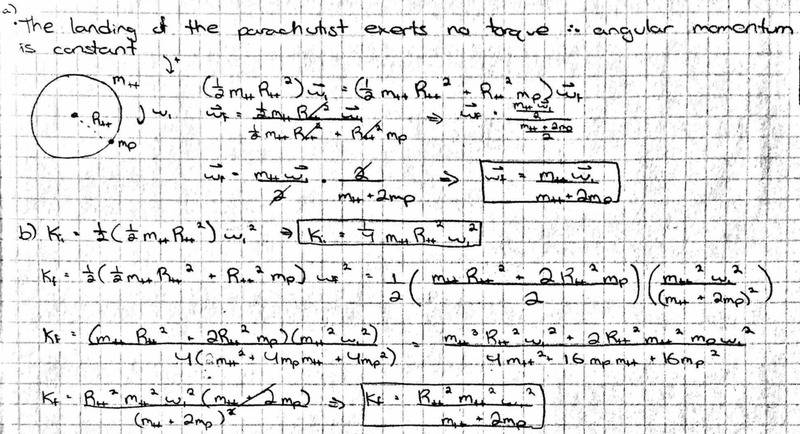# Angular momentum with a large wooden turntable

MITPhysics

## Homework Statement## Homework Equations

Li = Lf
L = I*omega
K = (1/2)*(I)*(omega)^2

## The Attempt at a Solution[/B]
Given that there are no non-conservative forces in action, I am assuming that the two kinetic energies should be equal. However, as shown by my work above, the two values are different, and I do not know where I went wrong.